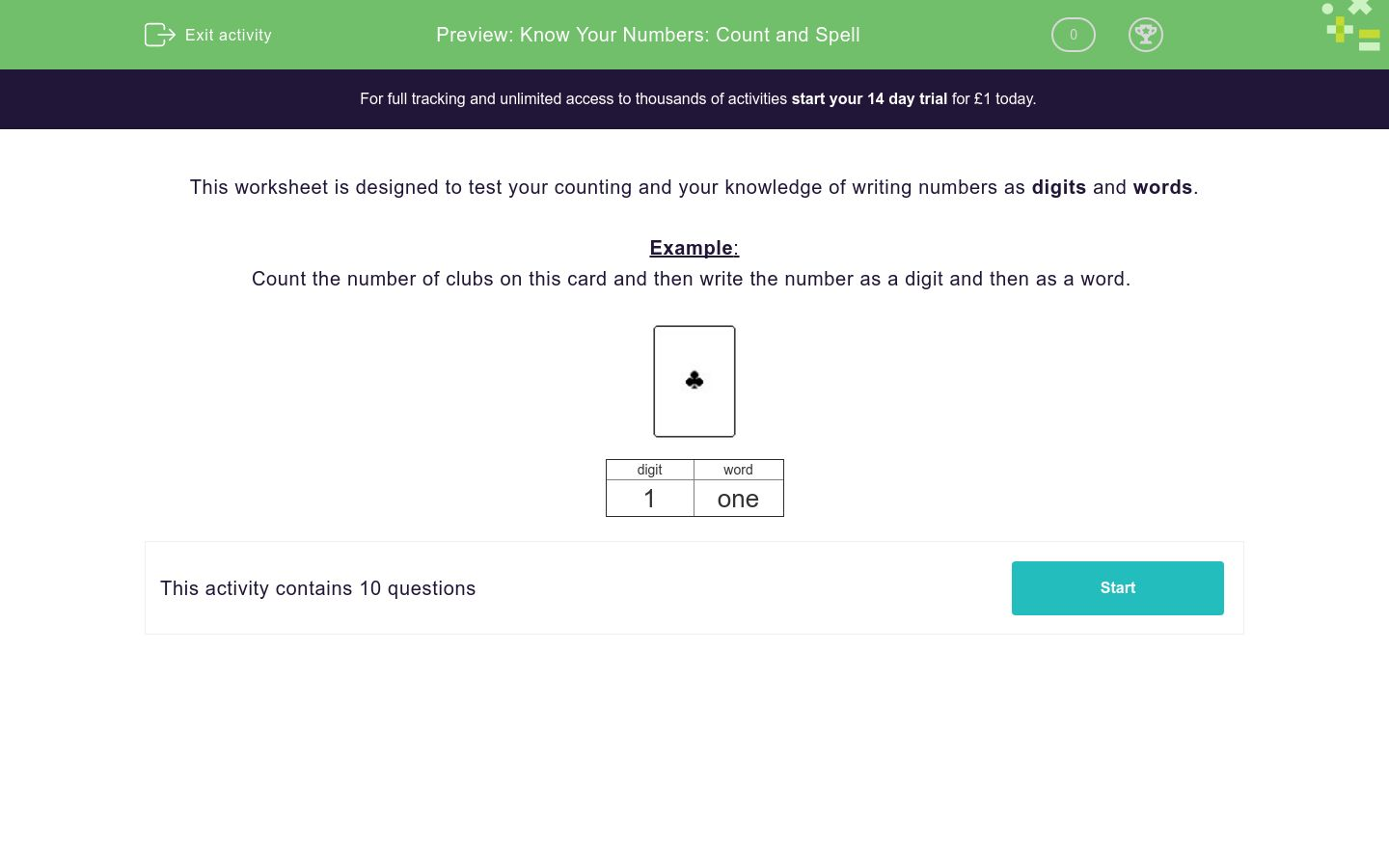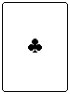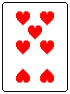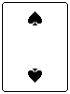# Know Your Numbers: Count and Spell

In this worksheets, students count the objects. Then they write the answer in both numbers and words.Key stage:  KS 1

Curriculum topic:   Number: Number and Place Value

Curriculum subtopic:   Read and Write Numerals to 100 (including multiples of 2, 5 and 10)

Difficulty level:### QUESTION 1 of 10

This worksheet is designed to test your counting and your knowledge of writing numbers as digits and words.

Example:

Count the number of clubs on this card and then write the number as a digit and then as a word.digit word 1 one

Count the number of hearts on this card and then write the number as a digit and then as a word.Count the number of spades on this card and then write the number as a digit and then as a word.Count the number of clubs on this card and then write the number as a digit and then as a word.Count the number of diamonds on this card and then write the number as a digit and then as a word.Count the number of spades on this card and then write the number as a digit and then as a word.Count the number of diamonds on this card and then write the number as a digit and then as a word.Count the number of spades on this card and then write the number as a digit and then as a word.Count the number of spades on this card and then write the number as a digit and then as a word.Count the number of spades on this card and then write the number as a digit and then as a word.Count the number of clubs on this card and then write the number as a digit and then as a word.• Question 1

Count the number of hearts on this card and then write the number as a digit and then as a word.EDDIE SAYS
7 = Seven
• Question 2

Count the number of spades on this card and then write the number as a digit and then as a word.EDDIE SAYS
5 = Five
• Question 3

Count the number of clubs on this card and then write the number as a digit and then as a word.EDDIE SAYS
4 = Four
• Question 4

Count the number of diamonds on this card and then write the number as a digit and then as a word.EDDIE SAYS
3 = Three
• Question 5

Count the number of spades on this card and then write the number as a digit and then as a word.EDDIE SAYS
8 = Eight
• Question 6

Count the number of diamonds on this card and then write the number as a digit and then as a word.EDDIE SAYS
10 = Ten
• Question 7

Count the number of spades on this card and then write the number as a digit and then as a word.EDDIE SAYS
9 = Nine
• Question 8

Count the number of spades on this card and then write the number as a digit and then as a word.EDDIE SAYS
2 = Two
• Question 9

Count the number of spades on this card and then write the number as a digit and then as a word.EDDIE SAYS
5 = Five
• Question 10

Count the number of clubs on this card and then write the number as a digit and then as a word.EDDIE SAYS
6 = Six
---- OR ----

Sign up for a £1 trial so you can track and measure your child's progress on this activity.

### What is EdPlace?

We're your National Curriculum aligned online education content provider helping each child succeed in English, maths and science from year 1 to GCSE. With an EdPlace account you’ll be able to track and measure progress, helping each child achieve their best. We build confidence and attainment by personalising each child’s learning at a level that suits them.

Get started